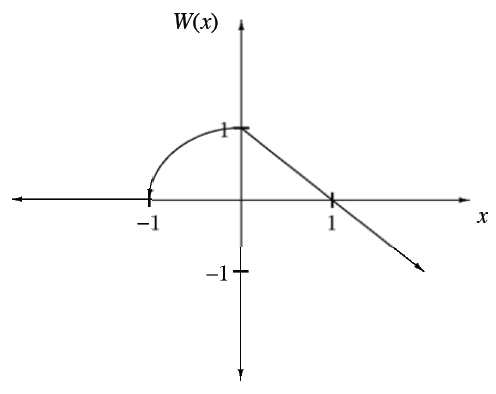Home > INT3 > Chapter Ch6 > Lesson 6.2.4 > Problem6-68

6-68.

A function $W(x)$ is sketched at right. Homework Help ✎

1. Make your own copy of the graph, and then sketch the graph of the inverse of $W(x)$.

Reflect the graph over the line $y=x$.

2. Is the inverse a function? Explain.

For every $x$-value, is there only one
corresponding $y$-value?